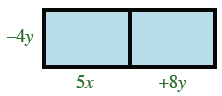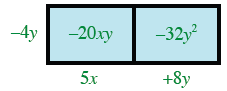Home > CCA > Chapter 3 > Lesson 3.3.1 > Problem3-81

3-81.

Find each of the following products by drawing and labeling a generic rectangle or by using the Distributive Property. Homework Help ✎

a.$−4y(5x+8y)$

• Make and label a generic rectangle.Fill in the boxes, and then add the boxes' labels together$−20xy−32y^2$

1. $9x(−4+10y)$

• Distribute the $9x$ over each of the terms in the parentheses.

$9x(−4)+9x(10y)$

Simplify.

$−36x+90xy=90xy−36x$

1. $(x^2−2)(x^2+3x+5)$

• Try using a generic rectangle to solve this problem.

$x^4+3x^3+3x^2−6x−10$